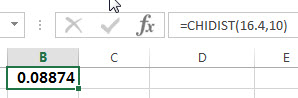# Excel CHIDIST function

This post will guide you how to use the CHIDIST function with syntax and examples in Microsoft excel.

### Description

The Excel CHIDIST function returns the right-tailed probability of the chi-squared distribution.

The CHIDIST function is a build-in function in Microsoft Excel and it is categorized as a Statistical Function.

The CHIDIST function is available in Excel 2016, Excel 2013, Excel 2010, Excel 2007, Excel 2003, Excel XP, Excel 2000, Excel 2011 for Mac.

### Syntax

The syntax of the CHIDIST function is as below:

`= CHIDIST (x,deg_freedom)`

Where the CHIDIST function arguments are:

• X -This is a required argument. The value at which you want to determine the chi-squared distribution.
• deg_freedom – This is a required argument.  The number of degrees of freedom. (the value must be an integer between 1 and 10^10)

Note:

• If any argument is non-numeric, the CHIDIST function will return #VALUE! Error.
• If deg_freedom argument is not integer and the CHIDIST function will truncate it.
• If x is negative, the CHIDIST function will return the #NUM! Error.
• If deg_freedom argument is smaller than 1 or greater than 10^10, the CHIDIST function will return the #NUM! Error.

### Excel CHIDIST Function Examples

The below examples will show you how to use Excel CHIDIST Function to get one-tailed probability of the chi-squared distribution.

1# to calculate the right-tailed probability of the chi-square distribution, using the following formula:

`=CHIDIST(16.4,10)`### Related Functions

• Excel CHIINV function
The Excel CHIINV function returns the inverse of the right-tailed probability of the chi-squared distribution.  For example, if the probability is equal to CHIDIST(x,…), then the CHIINV(probability,…) is equal to x.The syntax of the CHIINV function is as below:= CHIINV (probability, deg_freedom).…